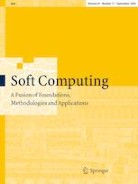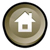Published:

# Algorithm for determining the mutual impact of nodes in weighted directed graphs

Dmytro Lande, Oleh Dmytrenko, Minglei Fu, Minchao Hu, Dmytro Manko & Andrei Snarskii

## Abstract

We propose an algorithm for computing the influence matrix and rank distribution of nodes of a weighted directed graph by calculating the nodes. mutual impact. The algorithm of accumulative impact solves problems of dimension and computational complexity arising in the analysis of large complex systems. The algorithm calculates the mutual impact of each pair of vertices, making it possible to rank the nodes according to their importance within the system and to determine the most influential components. It produces results similar to those of the commonly used impulse method when applied to graphs that are impulse-stable in an impulse process, while overcoming the disadvantages of the impulse method in other situations. Results are always obtained regardless of impulse stability; they do not depend on the initial impulse, so that the initial values of the weights affect the calculation results. When elements in the adjacency matrix of the weighted directed graph are multiplied by a constant factor, scale invariance is not violated, and the full affect for each of the nodes scales proportionally. Several examples of analyses of weighted directed graphs, including one related to the practical problem of urban solid waste removal, are provided to demonstrate the advantages of the proposed algorithm.